Test: Data Handling - 3

# Test: Data Handling - 3

Test Description

## 20 Questions MCQ Test Mathematics for Class 3: NCERT | Test: Data Handling - 3

Test: Data Handling - 3 for Class 3 2022 is part of Mathematics for Class 3: NCERT preparation. The Test: Data Handling - 3 questions and answers have been prepared according to the Class 3 exam syllabus.The Test: Data Handling - 3 MCQs are made for Class 3 2022 Exam. Find important definitions, questions, notes, meanings, examples, exercises, MCQs and online tests for Test: Data Handling - 3 below.
Solutions of Test: Data Handling - 3 questions in English are available as part of our Mathematics for Class 3: NCERT for Class 3 & Test: Data Handling - 3 solutions in Hindi for Mathematics for Class 3: NCERT course. Download more important topics, notes, lectures and mock test series for Class 3 Exam by signing up for free. Attempt Test: Data Handling - 3 | 20 questions in 40 minutes | Mock test for Class 3 preparation | Free important questions MCQ to study Mathematics for Class 3: NCERT for Class 3 Exam | Download free PDF with solutions
 1 Crore+ students have signed up on EduRev. Have you?
Test: Data Handling - 3 - Question 1

### DIRECTION: The given pictograph shows the number of 4 different kinds of plants Mohit bought. Study the pictograph and answer the questions.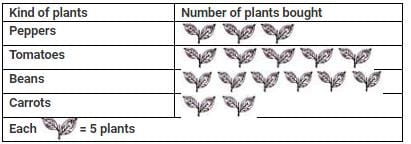Q. What is the total number of plants he buy?

Detailed Solution for Test: Data Handling - 3 - Question 1

Number of pepper plants he bought = 3×5=15
Number of tomato plants he bought = 5×5=25
Number of bean plants he bought = 6×5=30
Number of carrot plants he bought = 2×5=10
∴Total number of plants Mohit bought = 15+25+30+10 =80

Test: Data Handling - 3 - Question 2

### DIRECTION: The given pictograph shows the number of children who came to story hour each week. Study the pictograph and answer the following questions.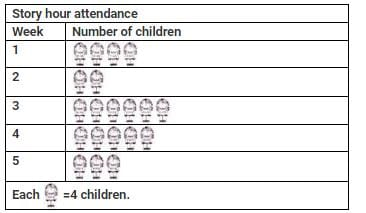Q. How many children came to story hour in week 4?

Detailed Solution for Test: Data Handling - 3 - Question 2

Number of children came in week 4 = 5 × 4 = 20

Test: Data Handling - 3 - Question 3

### DIRECTION: The given pictograph shows the number of children who came to story hour each week. Study the pictograph and answer the following questions.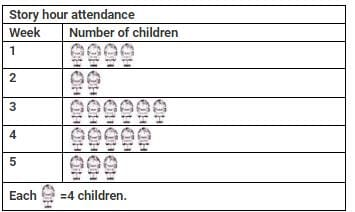Q. How many more children came for story hour in week 3 than in week 2?

Detailed Solution for Test: Data Handling - 3 - Question 3

Number of children came in week 3 = 6 × 4 = 24
Number of children came in week 2 = 2 × 4 = 8
∴ Difference = 24 − 8 = 16 So, 16 more children came for story hour in week 3 than in week 2.

Test: Data Handling - 3 - Question 4

DIRECTION: The given pictograph shows the number of children who came to story hour each week. Study the pictograph and answer the following questions.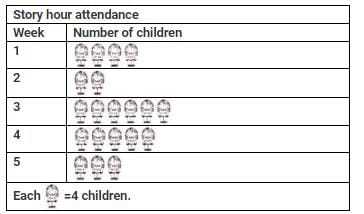Q. Total number of children came for story hours in all weeks is _______.

Detailed Solution for Test: Data Handling - 3 - Question 4

Number of children came in week 1 = 4×4=16
Number of children came in week 2 = 2×4=8
Number of children came in week 3 = 6×4=24
Number of children came in week 4 =5×4=20
Number of children came in week 5 =3×4=12
∴Total number of children came for story hour in all weeks =16+8+24+20+12=80

Test: Data Handling - 3 - Question 5

DIRECTION: The given pictograph shows the number of children who came to story hour each week. Study the pictograph and answer the following questions.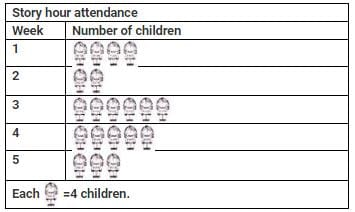Q. How many more children came to story hour in week 1 and week 5 together than in week 3?

Detailed Solution for Test: Data Handling - 3 - Question 5

Number of children came in week 3 = 24
Number of children came in week 1 and week 5 together =16+12=28 So, number of more children who came to story hour in week 1 and week 5 together than in week 3=28−24=4

Test: Data Handling - 3 - Question 6

Study the given table and answer the question based on the data in the table.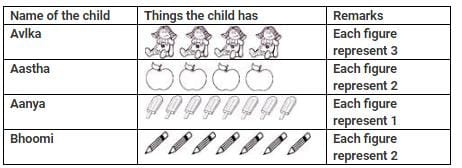Q. Who had the maximum number of items?

Detailed Solution for Test: Data Handling - 3 - Question 6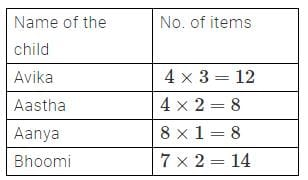∴ Bhoomi has the maximum number of items.

Test: Data Handling - 3 - Question 7

The given pictograph graph shows the number of bookmarks each student made. Study the pictograph and answer the following question.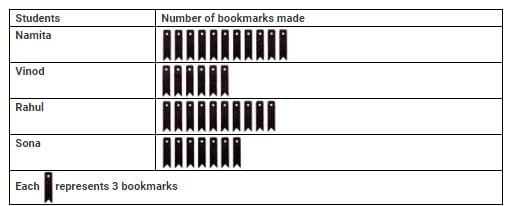Q. How many less bookmarks did Namita make than Sona and Vinod together?

Detailed Solution for Test: Data Handling - 3 - Question 7

Number of bookmarks made by Sona =7×3=21
Number of bookmarks made by Vinod = 6×3=18
∴ Number of bookmarks made by Sona and Vinod together =21+18=39
Now, number of bookmarks made by Namita =11×3=33
∴ Required difference =39−33=6. So, Namita made 6 less bookmarks than Sona and Vinod together.

Test: Data Handling - 3 - Question 8

The given graph shows the number of books read by pupils in a class from July to December. Study the given graph and answer the following question.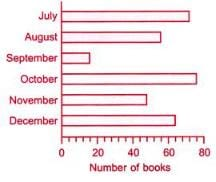Q. How many books were read altogether from July to November?

Detailed Solution for Test: Data Handling - 3 - Question 8

Number of books read in July = 72
Number of books read in August = 56
Number of books read in September =16
Number of books read in October = 76
Number of books read in November = 48
∴Total number of books read from July to November =72+56+16+76+48=268

Test: Data Handling - 3 - Question 9

The given pictograph shows the number of sweaters ordered of different size. Study the pictograph and answer the following question.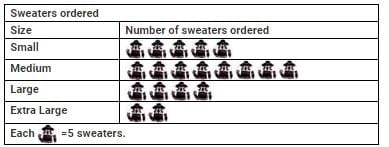Q. Which size of exactly 20 sweaters were ordered?

Detailed Solution for Test: Data Handling - 3 - Question 9

Number of large size sweaters ordered = 4 × 5 = 20
∴ large size sweaters were ordered exactly 20.

Test: Data Handling - 3 - Question 10

What is the total number of cellphones in the given figure: [Here 1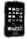= 100 cellphones]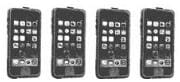Test: Data Handling - 3 - Question 11

Direction: A survey was conducted on a group of 90 students. The information obtained is shown below: [Here 1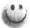= 10 persons]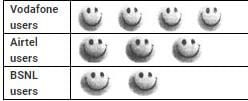Q. The number of vodafone users is ______

Test: Data Handling - 3 - Question 12

Direction: A survey was conducted on a group of 90 students. The information obtained is shown below: [Here 1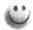= 10 persons]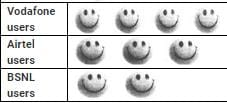Q. The number of Airtel users is _________

Test: Data Handling - 3 - Question 13

Direction: A survey was conducted on a group of 90 students. The information obtained is shown below: [Here 1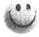= 10 persons]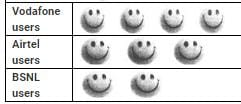Q. The number of BSNL users is __________

Test: Data Handling - 3 - Question 14

If each of the following balls is arranged in a group of 5 balls, how many such groups are possible?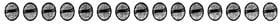Test: Data Handling - 3 - Question 15

Find the total number of tigers in the given figure? [Here 1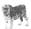= 6 tigers]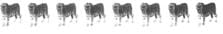Test: Data Handling - 3 - Question 16

If * represents 12 pens then which one of the following will represents 72 pens?

Test: Data Handling - 3 - Question 17

Direction: Read the table given below and answer the question accordingly: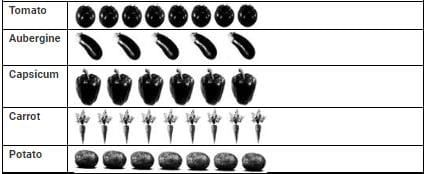Q. How many capsicums are there?

Test: Data Handling - 3 - Question 18

Direction: Read the table given below and answer the question accordingly: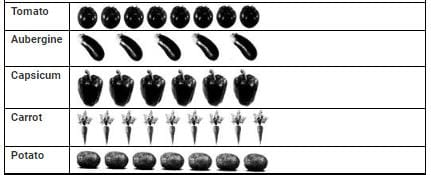Q. Which kind of vegetable is minimum in number?

Test: Data Handling - 3 - Question 19

Direction: Read the table given below and answer the question accordingly: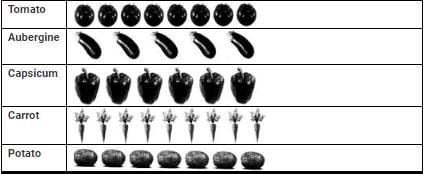Q. Which kind of vegetable is maximum in number?

Test: Data Handling - 3 - Question 20

Direction: Read the table given below and answer the question accordingly: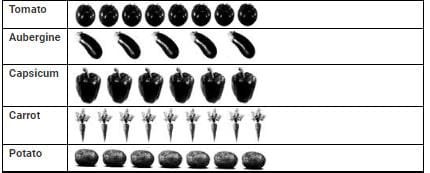Q. What is the total number of vegetables?

## Mathematics for Class 3: NCERT

51 videos|84 docs|44 tests
 Use Code STAYHOME200 and get INR 200 additional OFF Use Coupon Code
Information about Test: Data Handling - 3 Page
In this test you can find the Exam questions for Test: Data Handling - 3 solved & explained in the simplest way possible. Besides giving Questions and answers for Test: Data Handling - 3, EduRev gives you an ample number of Online tests for practice

## Mathematics for Class 3: NCERT

51 videos|84 docs|44 tests